# Geometry

 1 Introduction to Geometry 2 Euclid's Geometry 3 Circles 4 Triangles 5 Lines 6 Angles 7 Quadrilaterals 8 Solid Shapes 9 3D Shapes 10 Coordinate Geometry 11 Vectors 12 FREE Worksheets of Geometry

## Introduction

Geometry (from the Ancient Greek: γεωμετρία; geo- "earth", -metron "measurement") is a branch of mathematics that is primarily concerned with questions pertaining to:

• the shape of figures
• the size of figures
• the relative position of figures
• the properties of space

You can go ahead and explore all important topics in Geometry by selecting the topics from this list below:

 Euclid's Geometry Quadrilaterals Circles Solid Shapes Triangles 3D shapes Lines Coordinate Geometry Angles Vectors

## Euclid’s Geometry

Euclidean Geometry refers to the study of plane and solid figures on the basis of axioms (a statement or proposition) and theorems employed by the Greek mathematician Euclid, who is often referred to as the “Father of Geometry”.

There are $$5$$ basic postulates of Euclidean Geometry

Euclid was one of the greatest thinkers of all time. We study Euclidean geometry to understand the fundamentals of geometry. In this section, you will learn about the different concepts of Euclidean geometry like Points and Lines, Axioms and Postulates, Euclid’s Axioms and Postulates, Geometrical Proof, and Euclid’s Fifth Postulate. Euclid’s Geometry is introduced to students in Grade 9.

## Circles

A circle is a closed figure and has no edges or corners. It is defined as the set of all points in a plane that are equidistant from a given point called the center of the circle.

Example: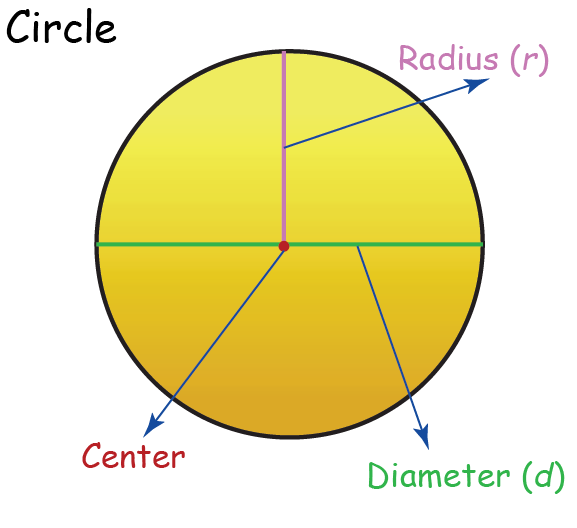Circles are introduced in Grade 6.

In this topic, we cover various topics related to circle starting with the definition of a circle by first understanding What is a Circle? You will learn how to find the Ratio of Circumference to Diameter, how to measure the Area of the Circle, the concept of Concentric Circles, Chords and Diameters, the Perpendicular Bisector of a Chord, and the Symmetry of any Circle. You can also learn how to Construct Circles, what are Equal and Unequal Chords, Arcs and Subtended Angles, Concyclic Points, Cyclic Quadrilaterals. We also define tangents by helping you understand What is a Tangent and its related concepts like Tangent as a Special Case of Secant, Uniqueness of the Tangent at a Point, Tangents from an External Point, Circles touching Each Other, Alternate Segment Theorem, and Common Tangents.

## Triangles

A  triangle is a closed figure with three sides and three vertices.

Example: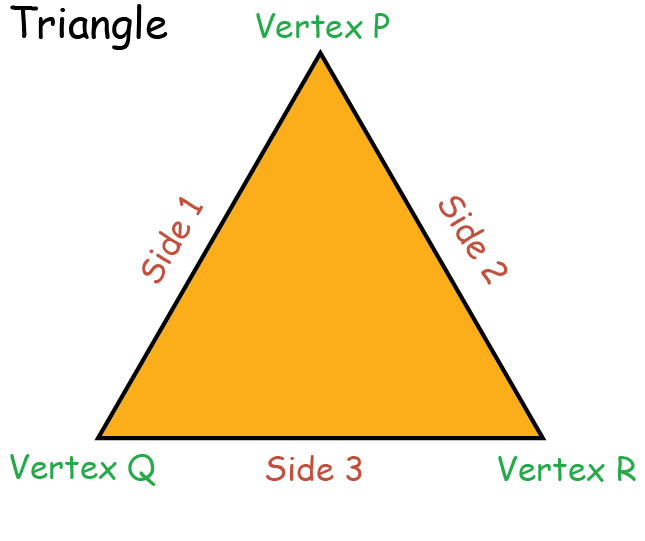Triangles are introduced in Grade 7.

Now that you are familiar with the definition of a triangle, let's get you introduced to different concepts related to the triangle such as What is Congruence, Congruence in Triangles, The SAS Criterion, Is there an SSA Criterion, Perpendicular Bisectors, The ASA Criterion, The ASA Criterion Proof, Is There an AAS Criterion, What is an Isosceles Triangle, The SSS Criterion, The SSS Criterion - Proof, The RHS Criterion, The RHS Criterion - Proof.

You will also get to learn more concepts like Relative Magnitudes of Sides and Angles, The Triangle Inequality, Distance of a point from a line, Angle Bisector, Triangles - Same Base, Same Parallels, Pythagoras Theorem, Triangle Areas - Basic Calculations, Heron's Formula, Basic Triangle Constructions, Advanced Triangle Constructions, Angle Sum Property, Proof of the Angle Sum Property, Exterior Angle Theorem, What is Similarity, The Similarity in Triangles, Basic Proportionality Theorem, Internal Division, Angle Bisector Theorem, AA Criterion in Triangles, SSS Criterion in Triangles, SAS Criterion in Triangles, Areas of Similar Triangles and the Pythagoras Theorem.

## Lines

A line is a set of points that extends in two opposite directions. Both ends of a line can be extended indefinitely. A line has no endpoints.

Example: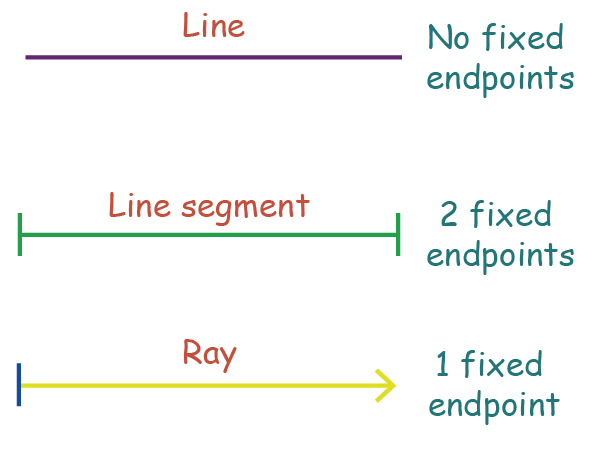Lines are introduced in Grade 3.

We all know what a line is. It is one of the basic concepts of geometry. But often students mix line with line segments and ray! In this topic, you will learn how to differentiate a line, line segment, and ray. We also cover the concept of intersecting and non-intersecting lines.

## Angles

When two straight lines intersect at a point, they form an angle. Angles are usually measured in degrees.

Example: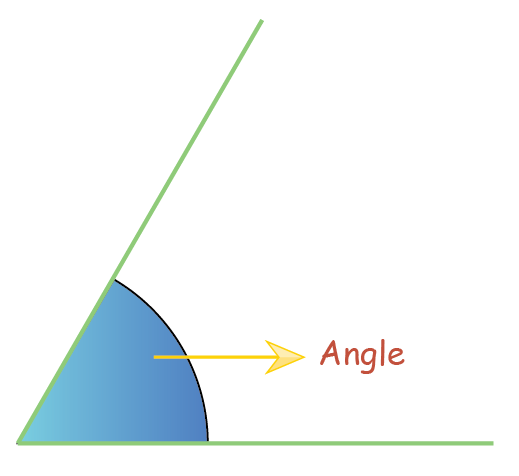Angles are introduced in Grade 4.

Now that you know what an angle is, let us explore more about it. In this section, we will cover concepts like Pairs of Angles, Transversals and Related Angles, Corresponding Angles, Interior Angles, Lines Parallel to the Same Line, Essence of Geometrical Constructions which include How to use a Protractor, Constructing Angle Bisectors, Constructing Perpendicular Bisectors, Constructing an Angle of 90°, Constructing an Angle of 60° and Constructing Perpendicular from Point to Line.

A quadrilateral is a polygon with $$4$$ sides and $$4$$ vertices or corners.

Example: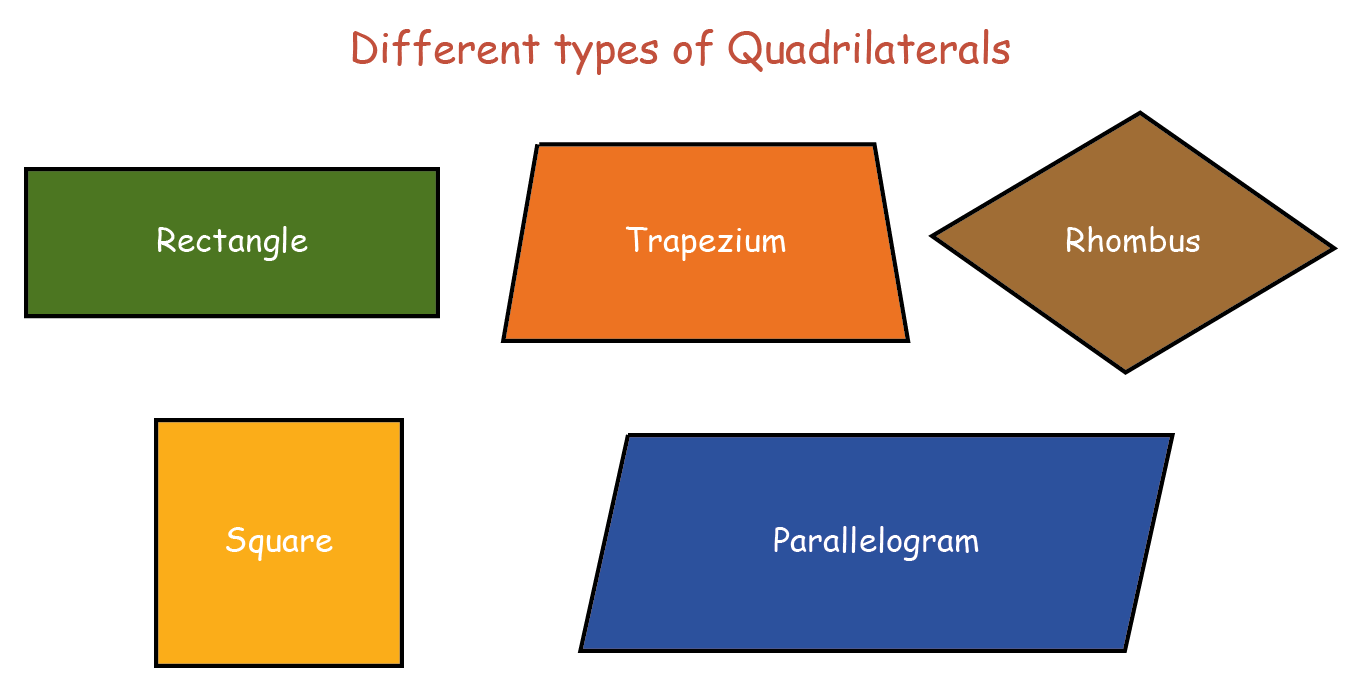Did you know? The word Quad means four! And now you also know that a quadrilateral has four edges and four corners. A quadrilateral can be of different shapes, let’s dive in and explore more about quadrilaterals. We will be covering topics such as Angle Sum Property in Quadrilaterals, Some Particular Types of Quadrilaterals, Parallelograms, Properties of Parallelograms, Mid-Point Theorem, Basic Area Concepts, Same Base Same Parallels, Parallelograms - Same Base, Same Parallels and Heron's Formula and Quadrilaterals.

## Solid Shapes

Solid shapes are three-dimensional in nature. The three dimensions that are taken into consideration are:

• Length
• Height

Example: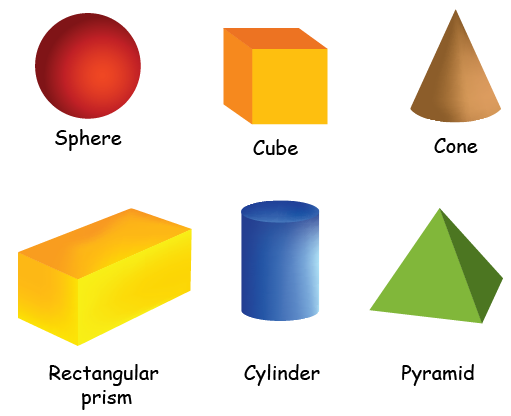Solid Shapes are introduced in Grade 2.

Solid shapes are different from plain shapes. Solid figures can also be called 3D figures. There are different types of solid figures like a cylinder, cube, sphere, cone, etc and these figures acquire some space, unlike the 2D figures. In this section, we will learn various concepts about symmetry such as Line of Symmetry, Rotational Symmetry, Order of Symmetry and Mirror Symmetry.

## 3D Shapes

In geometry, 3D Shapes are the solid objects that has three dimensions- length, width and height. Unlike flat 2D shapes like squares and rectangles , 3-D shape has depth or thickness. Some real life examples of 3 D shapes are rubik’s cube, ice cream cone,or an orange.

3D shapes are introduced in Grade 6.

## Coordinate Geometry

Coordinate Geometry is a branch of geometry where the position of any given point on a plane is defined with the help of an ordered pair of numbers, or coordinates.

Example: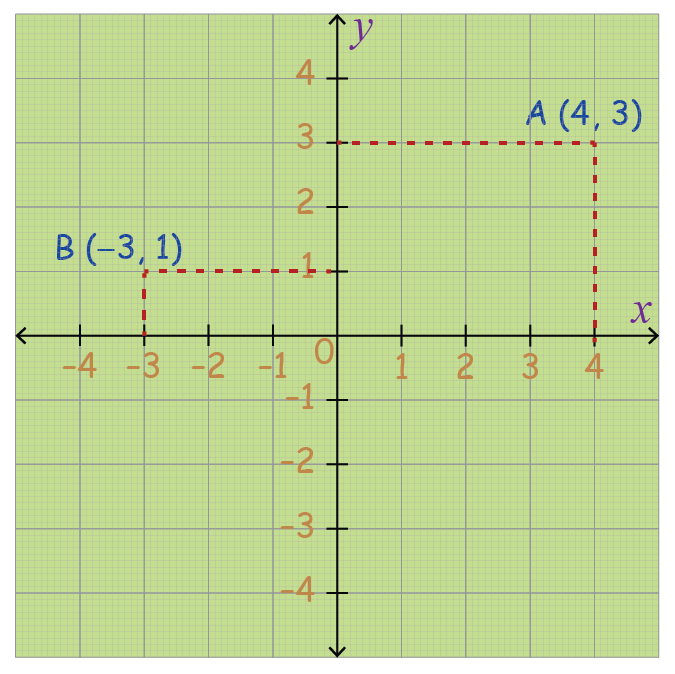In the above example, the point $$\text{A}$$ is defined as $$(4,3).$$ Point $$\text{B}$$ is defined as $$(-3,1).$$

Coordinate Geometry is introduced in Grade 9.

It is a fun and interesting concept of mathematics. In coordinate geometry, we use a graph and plot points along the x-axis and y-axis. Let's go ahead and understand the concepts of coordinate geometry which includes topics starting from the basics such as Labelling Points on a Number Line, Labelling Points in a Plane, Cartesian System, Straight Line Distance, The Distance Formula, Internal Division, External Division, Area of a Triangle, Area of a Quadrilateral, Collinearity, Lines parallel to the Axes, Slope-Intercept Form of a Line, Point-Slope Form, Two-Point Form, General Equation of a Line, Intersection of Two Lines, Circles Centered at the Origin, Circles with Arbitrary Centers and General Equations of a Circle.

## Vectors

An object that has both magnitude and direction is referred to as a vector. Geometrically, it can be visualized as a directed line segment: the length of the line segment becomes the magnitude of the vector and the arrow indicates the direction of the vector.

Example: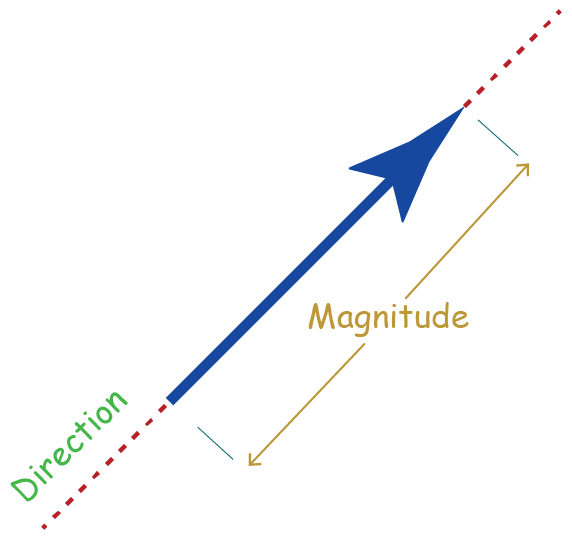Vectors are introduced in Grade 10.

Now that you are familiar with what a vector is, you can visualize the direction and magnitude of a vector easily. Vector quantities are often represented by scaled vector diagrams. There is a lot more to learn about vectors. We will cover topics such as Vector Addition as Net Effect, Adding Two Vectors in General, Subtracting Two Vectors, The Triangle Inequality, Multiplication of a Vector by a Scalar, The i-j system, Resolving a Vector into Components and Handling Vectors Specified in the i-j form.

## Worksheets

Lines and plane shapes
Lines and plane shapes
Polygons and Circles
Polygons and Circles
Geometry
Geometry
grade 9 | Questions Set 1
Geometry
grade 9 | Questions Set 2
Geometry